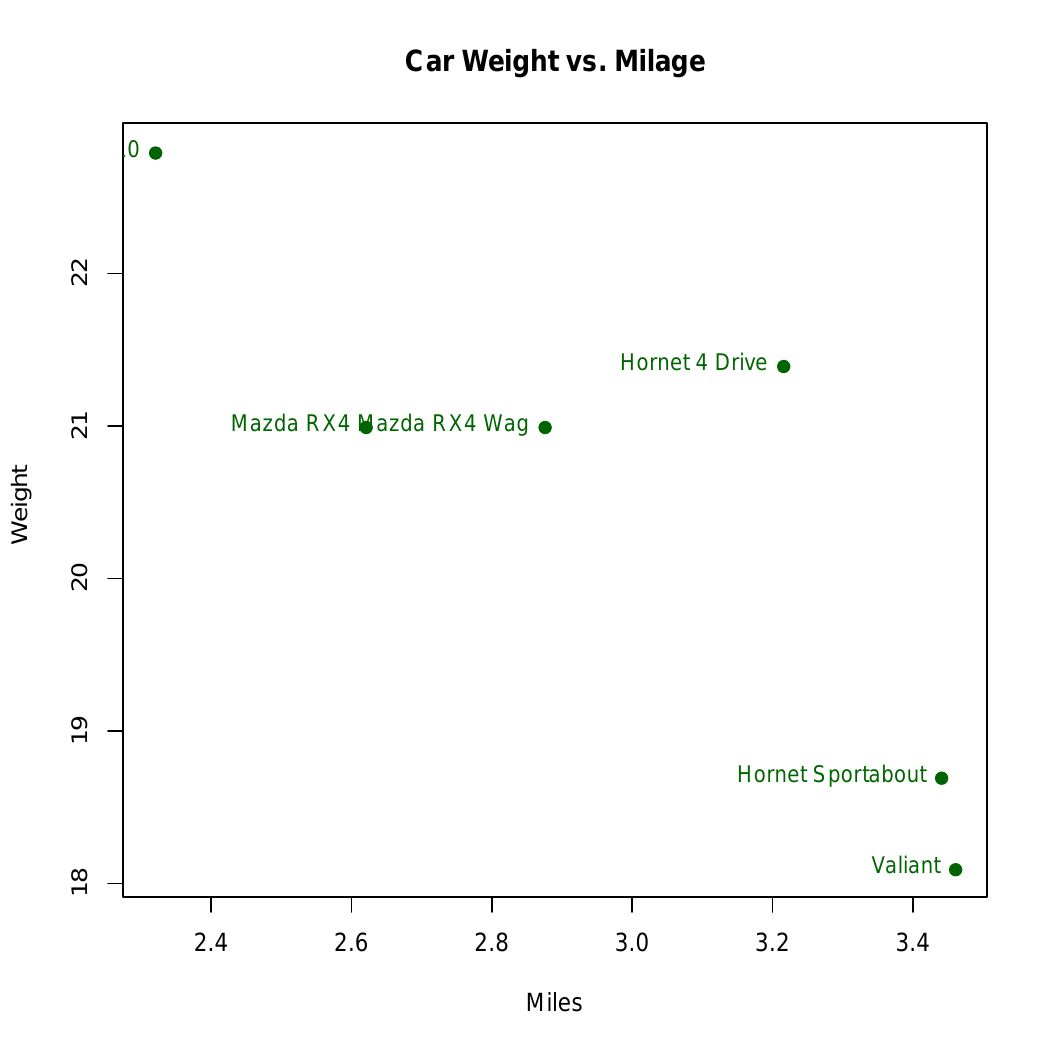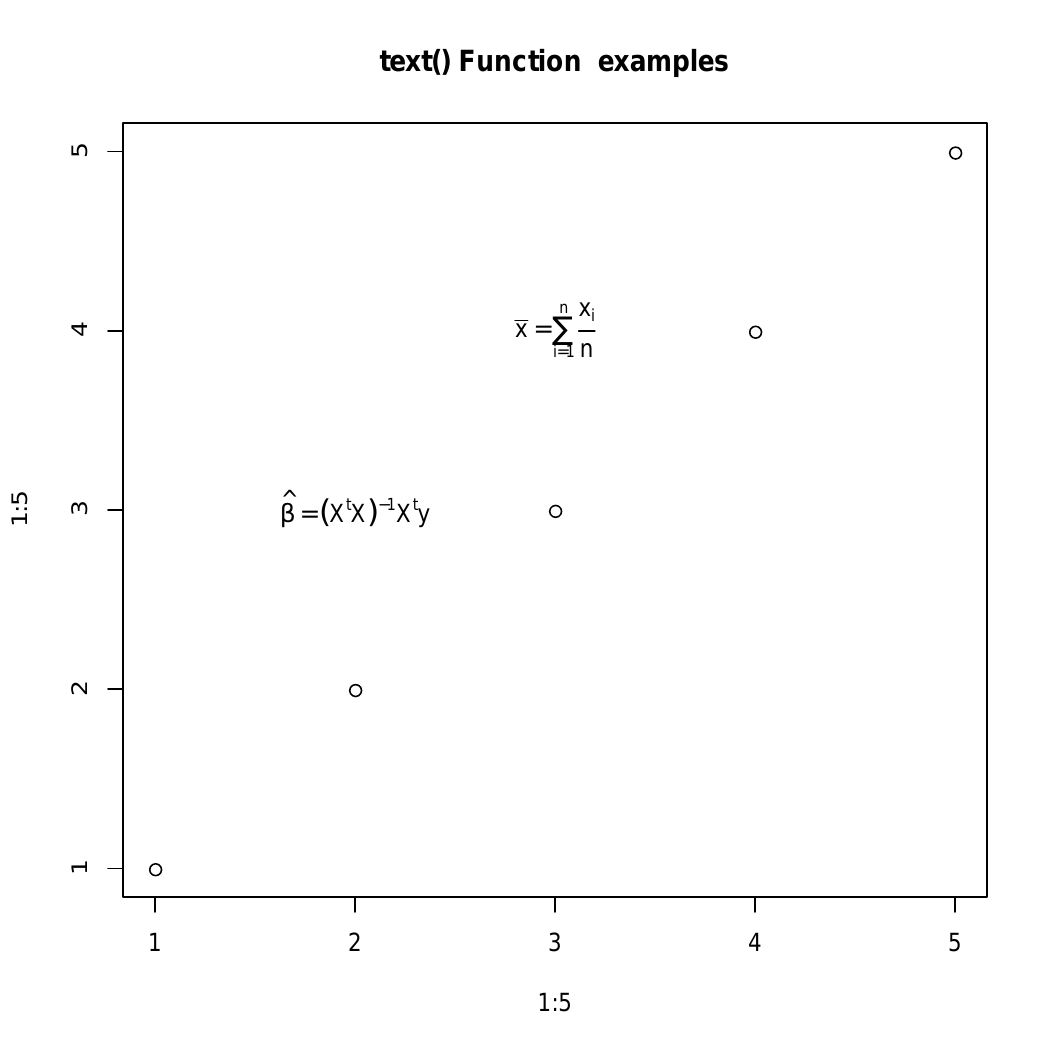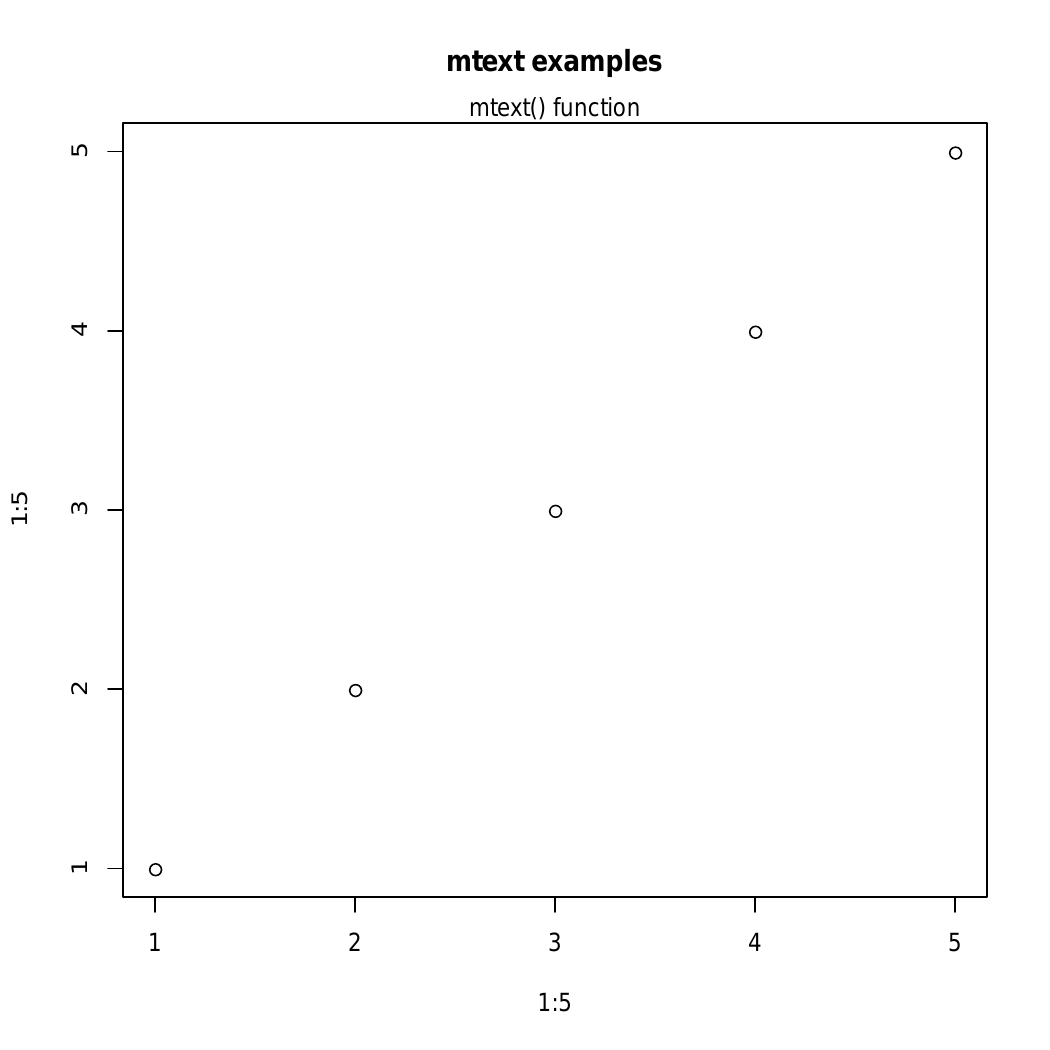# Adding Text to Plots in R programming – text() and mtext () Function

Text is defined as way to describe data related to graphical representation. They work as a labels to any pictorial or graphical representation. In this article we will learn to to add a text to a plot in R Language by using the `text()` and `mtext()` functions.

Syntax: text(x, y, labels)

Parameters:
x and y: numeric values specifying the coordinates of the text to plot
labels: the text to be written

Example 1:

 `# R program to add text to plot ` ` `  `# Calling data set ` `d<-``head``(mtcars) ` ` `  `# Plotting the graph ` `plot``(d[, ``'wt'``], d[, ``'mpg'``],  ` `     ``main = ``" Car Weight vs. Milage "``, ` `     ``xlab = ``"Miles"``, ylab = ``" Weight"``, ` `     ``pch = 19, col = ``"darkgreen"``) ` ` `  `# Calling text() function ` `text``(d[, ``'wt'``], d[, ``'mpg'``],  ``row.names``(d), ` `     ``cex = 0.88, pos = 2, col = ``"darkgreen"``) `

Output:In above example, the text is added to plot of ‘mtcar’ dataset.

Example 2: Implementation of text() to add mathematical annotation to a plot

 `# R program to add text to plot ` ` `  `# Plotting the graph ` `plot``(1:5, 1:5,  ` `     ``main = ``"text() Function  examples"``) ` ` `  `# Calling text() function ` `text``(2, 3, ``expression``(``hat``(beta) == (X^t * X)^{-1} * X^t * y)) ` `text``(3, 4, ``expression``(``bar``(x) == ``sum``(``frac``(x[i], n), i==1, n))) `

Output :In above example the the `text()` function is used to add mathematical annotation to a plot

##### Add text to the margins of the graph

`mtext()` function is used to add text to the margins of the plot.

Syntax: mtext(text, side)

Parameters:
text: text to be written
side: An integer specifying the side of the plot, such as: bottom, left, top, and right.

Returns: Added text in the margins of the graph

Example:

 `# R program to add text to a plot ` ` `  `# Creating a plot ` `plot``(1:5, 1:5,  ` `     ``main = ``"mtext examples"``) ` ` `  `# Calling mtext() function ` `mtext``(``"mtext() function"``, side = 3) `

Output:Here, in above example the side specifies the side of the plot such as bottom, left, top, right . And in the given the side=3 i.e the top part of the plot

My Personal Notes arrow_drop_upCheck out this Author's contributed articles.

If you like GeeksforGeeks and would like to contribute, you can also write an article using contribute.geeksforgeeks.org or mail your article to contribute@geeksforgeeks.org. See your article appearing on the GeeksforGeeks main page and help other Geeks.

Please Improve this article if you find anything incorrect by clicking on the "Improve Article" button below.

Article Tags :

Be the First to upvote.

Please write to us at contribute@geeksforgeeks.org to report any issue with the above content.